# Signal Processing

Any information about a quantity, any measurement, any time varying function can be perceived and analyzed as a signal.

This post aims to inform the reader about the mathematical and physical signal processing, for quantities mostly used in electrical engineering.

Consider the arbitrary signal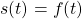As can be seen in figure below, an AC signal has zero mean value. In electrical engineering, a DC signal is not necessarily constant. It can include AC component (ripple) as long as its amplitude is smaller than its DC.

Using Fourier Series, it can be analyzed as

(1)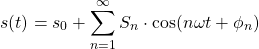Equation (1) is valid for any periodic signal, but it is possible to use the Fourier Series in any non-periodic signal just by defining a new subdomain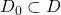, where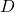is the domain of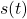.

DC or Mean Value of a Signal

By definition, the mean value ofis presented in equation (2).

(2)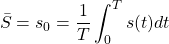which, not coincidentally, is the same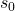as in Fourier Series.

RMS of Signal

“Root Mean Square” value is the “equivalent DC value of an sinusoidal signal”, meaning that is equal to the value of the direct current that would produce the same average power dissipation in a resistive load. By definition is

(3)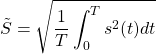If Fourier Series is already available, we can calculate the RMS value as

(4)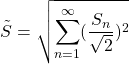or more simply as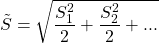Ifhas a non-zero DC component

(5)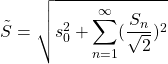Review